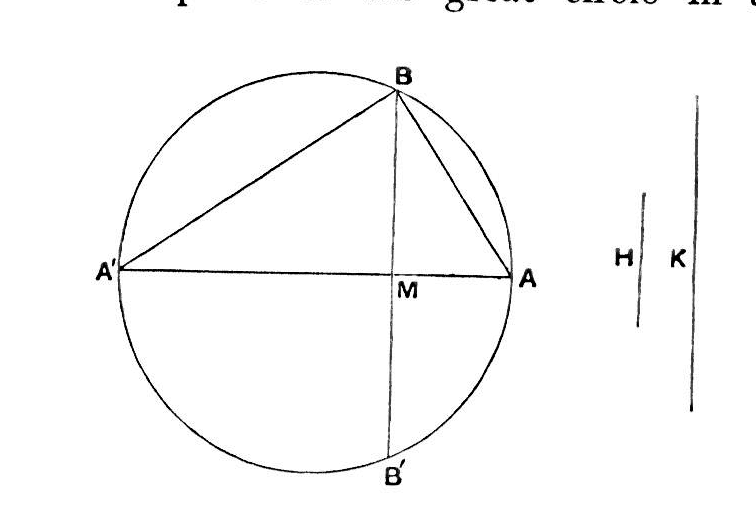Proposition 3. (Problem.)

To cut a given sphere by a plane so that the surfaces of the segments may have to one another a given ratio.Suppose the problem solved. Let AA' be a diameter of a great circle of the sphere and suppose that a plane perpendicular to AA' cuts the plane of the great circle in the straight line BB', and AA' in M, and that it divides the sphere so that the surface of the segment BAB'  has to the surface of the segment BA'B'  the given ratio.

Now these surfaces are respectively equal to circles with radii equal to AB, A'B [I. 42, 43].
Hence the ratio AB2 : A'B2 is equal to the given ratio, i.e. AM is to MA' in the given ratio.
Accordingly the synthesis proceeds as follows.

If H: K be the given ratio, divide AA' in M so that

AM: MA'=H: K.
Then AM: MA'=AB2 : A'B2